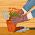## Saturday, August 8, 2009

### Trend Analysis with the Cox-Stuart test in R

The Cox-Stuart test is defined as a little powerful test (power equal to 0.78), but very robust for the trend analysis. It is therefore applicable to a wide variety of situations, to get an idea of the evolution of values obtained. The proposed method is based on the binomial distribution. In R there is no function to perform a test of Cox-Stuart, so now we see the logical steps that are the basis of test and finally we can write the function ourself.

You want to assess whether there is an increasing or decreasing trend of the number of daily customers of a restaurant. We have the number of customers in 15 days:
Customers: 5, 9, 12, 18, 17, 16, 19, 20, 4, 3, 18, 16, 17, 15, 14

To perform the test of Cox-Stuart, the number of observations must be even. In our case we have 15 observations. Delete, therefore, the observation at position (N+1)/2 (here the observation with value = 20):

`customers = c(5, 9, 12, 18, 17, 16, 19, 20, 4, 3, 18, 16, 17, 15, 14)length(customers) 15cust_even = customers[ -(length(customers)+1)/2 ]length(cust_even) 14`

Now we have 14 observations, and we can then proceed. Divide the observations into two vectors, the first containing the first half of the measures, and the second the second half:

`fHalf = cust_even[1:7]sHalf = cust_even[8:14]fHalf  5  9 12 18 17 16 19sHalf  4  3 18 16 17 15 14`

Now subtract, value by value, the content of the two vectors:

`difference = fHalf - sHalfdifference  1  6 -6  2  0  1  5`

Now consider only the signs of the contents of the vector difference

`signs = sign(difference)signs  1  1 -1  1  0  1  1`

A difference has value 0 and therefore also in the vector with the signs there is a value equal to 0. This must be eliminated:

`signs = signs[ signs != 0 ]signs  1  1 -1  1  1  1`

We obtained six differences, and then six signs. Now we have to count the number of positive-signs and the number of negative-signs:

`pos = signs[signs > 0]neg = signs[signs < 0]length(pos) 5length(neg) 1`

Now we choose the number of signs that is smaller. In this case we choose the number of negative signs (1). We compute the probability to obtain x = 1 successes on N = 6 experiments, each of which yields success with probability p = 0.5 (binomial distribution):

`pbinom(1, 6, 0.5) 0.109375`

The value so calculated is higher than 0.05 (we choose a significance level of 95%). Therefore there is no significant trend (which would have been in decline since the number of negative signs is minor).
If the value was less than 0.05, we accepted the hypothesis of a significant trend.

Now try to fit a regression model, and observe the p-value of the slope: the coefficient b is not significant.

`customers = c(5, 9, 12, 18, 17, 16, 19, 20, 4, 3, 18, 16, 17, 15, 14)days <- c(1:length(customers))model <- lm(customers ~ days)summary(model)Call:lm(formula = customers ~ days)Residuals:    Min      1Q  Median      3Q     Max -11.090  -2.173   1.352   3.967   6.467 Coefficients:            Estimate Std. Error t value Pr(>|t|)   (Intercept)  11.3048     3.1104   3.634  0.00303 **days          0.2786     0.3421   0.814  0.43014   ---Signif. codes:  0 ‘***’ 0.001 ‘**’ 0.01 ‘*’ 0.05 ‘.’ 0.1 ‘ ’ 1 Residual standard error: 5.724 on 13 degrees of freedom`

Here is the code to perform a Cos-Stuart test, written by me.

`cox.stuart.test =function (x){  method = "Cox-Stuart test for trend analysis"  leng = length(x)  apross = round(leng) %% 2  if (apross == 1) {    delete = (length(x)+1)/2    x = x[ -delete ]   }  half = length(x)/2  x1 = x[1:half]  x2 = x[(half+1):(length(x))]  difference = x1-x2  signs = sign(difference)  signcorr = signs[signs != 0]  pos = signs[signs>0]  neg = signs[signs<0]  if (length(pos) < length(neg)) {    prop = pbinom(length(pos), length(signcorr), 0.5)    names(prop) = "Increasing trend, p-value"    rval <- list(method = method, statistic = prop)    class(rval) = "htest"    return(rval)  }  else {    prop = pbinom(length(neg), length(signcorr), 0.5)    names(prop) = "Decreasing trend, p-value"    rval <- list(method = method, statistic = prop)    class(rval) = "htest"    return(rval)  }}`

We can now use the function just created:

`customers = c(5, 9, 12, 18, 17, 16, 19, 20, 4, 3, 18, 16, 17, 15, 14)cox.stuart.test(customers)        Cox-Stuart test for trend analysisdata:  Decreasing trend, p-value = 0.1094`

1.How can I cite you?
I'm using your code for the Stuart-Cox in an Article.

2.Hi Elisa
really thanks for your decision to cite my work!
You can use either the APA style or MLA style for citation:

APA style
MARTINO, Tommaso (2009). Trend analysis with the Cox-Stuart test in R. Retrieved 2010-02-17, from <http://statistic-on-air.blogspot.com/2009/08/trend-analysis-with-cox-stuart-test-in.html>

MLA style
MARTINO, Tommaso. Trend analysis with the Cox-Stuart test in R. <http://statistic-on-air.blogspot.com/2009/08/trend-analysis-with-cox-stuart-test-in.html>. August 2009. Web. 17 February 2010

Choose which you prefer, and/or modify it in according with your guidelines.
Can you please link me the article when it is published?
Tommaso

3.Hi,
How the code would look like if the observations were higher than 20?

4.Hi,
Congratulations for your work.
I don't understand "The value so calculated is less than 0.05 (we choose a significance level of 95%). Therefore there is no significant trend (which would have been in decline since the number of negative signs is minor).
If the value was less than 0.05, we accepted as a significant the trend."
The value calculated is over 0.05 (0.10). therefore there is significant trend. Or not?

5.6.HI the trend analysis is important to tes in R thanks bye.

7.how to run this test from command line (CMD)